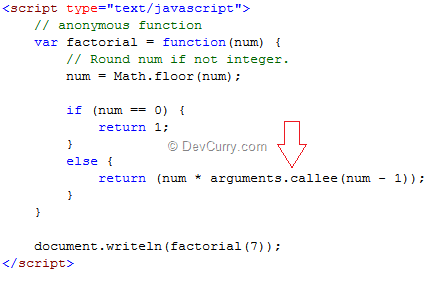# JavaScript: Recursive Anonymous Function

 Tweet
I recently saw a question on the forums – How to make a JavaScript anonymous function refer to itself? In other words, how to make an anonymous function in JavaScript call itself from within itself.

The answer is by using arguments.callee.

Let’s see an example. First here’s an example of a recursive function that is not anonymous.

```<script type="text/javascript">
function factorial(num) {
// Round num if not integer.
num = Math.floor(num);

if (num == 0) {
return 1;
}
else {
return (num * factorial(num - 1));
}
}

document.writeln(factorial(7));
</script>
```

The code shown above returns 5040.

Now if the same were to be written as an anonymous function and called recursively, we will use arguments.callee as shown below:Output remains the same, i.e. 5040.

Note: arguments.callee cannot be defined in “strict” mode and it can behave slow at times.